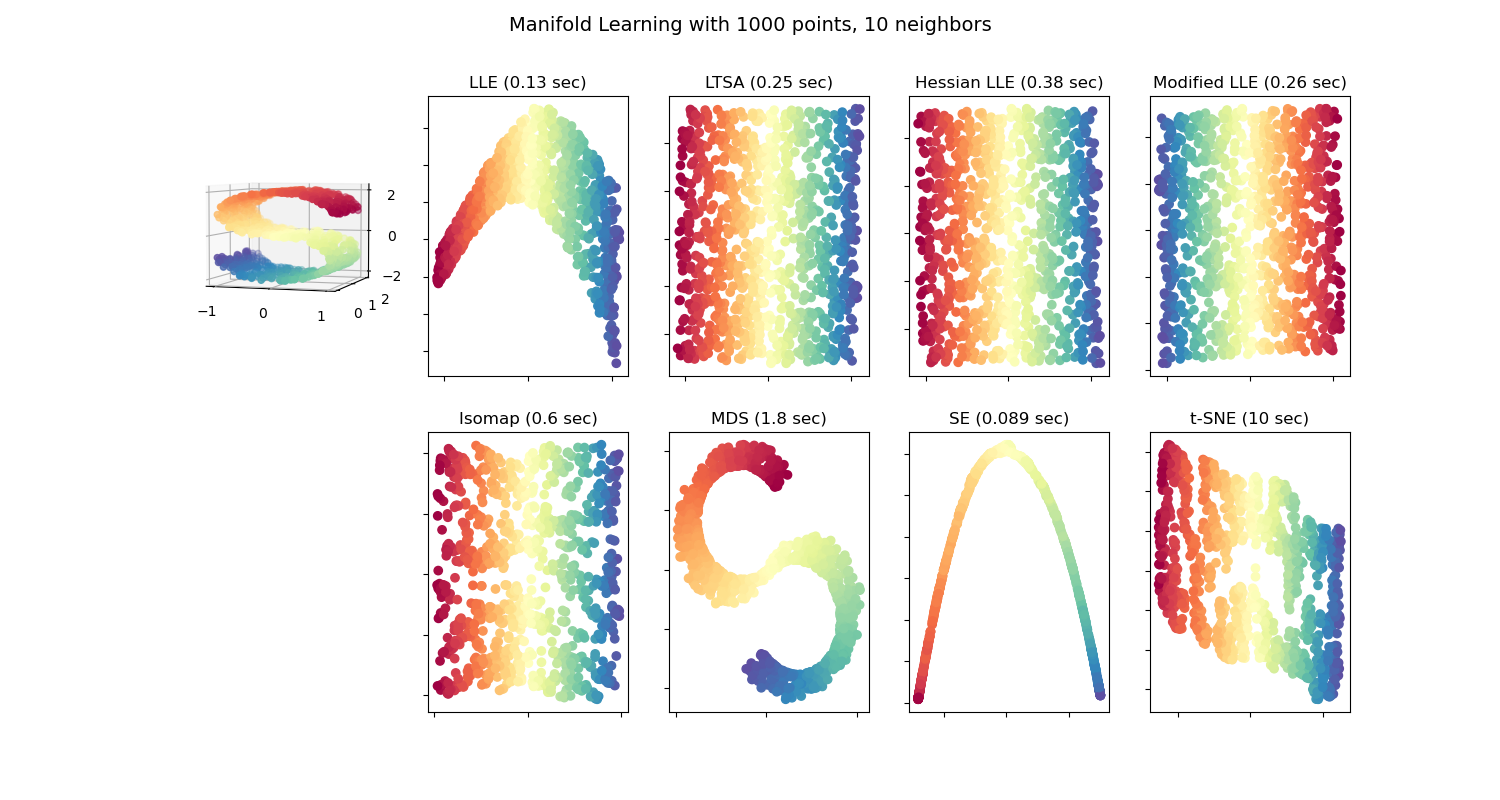# Comparison of Manifold Learning methods¶

An illustration of dimensionality reduction on the S-curve dataset with various manifold learning methods.

For a discussion and comparison of these algorithms, see the manifold module page

For a similar example, where the methods are applied to a sphere dataset, see Manifold Learning methods on a severed sphere

Note that the purpose of the MDS is to find a low-dimensional representation of the data (here 2D) in which the distances respect well the distances in the original high-dimensional space, unlike other manifold-learning algorithms, it does not seeks an isotropic representation of the data in the low-dimensional space.Out:

/home/circleci/project/sklearn/utils/validation.py:70: FutureWarning: Pass n_neighbors=10, n_components=2 as keyword args. From version 1.0 (renaming of 0.25) passing these as positional arguments will result in an error
warnings.warn(f"Pass {args_msg} as keyword args. From version "
LLE: 0.13 sec
LTSA: 0.25 sec
Hessian LLE: 0.38 sec
Modified LLE: 0.26 sec
Isomap: 0.6 sec
MDS: 1.8 sec
SE: 0.089 sec
t-SNE: 10 sec


# Author: Jake Vanderplas -- <vanderplas@astro.washington.edu>

print(__doc__)

from collections import OrderedDict
from functools import partial
from time import time

import matplotlib.pyplot as plt
from mpl_toolkits.mplot3d import Axes3D
from matplotlib.ticker import NullFormatter

from sklearn import manifold, datasets

# Next line to silence pyflakes. This import is needed.
Axes3D

n_points = 1000
X, color = datasets.make_s_curve(n_points, random_state=0)
n_neighbors = 10
n_components = 2

# Create figure
fig = plt.figure(figsize=(15, 8))
fig.suptitle("Manifold Learning with %i points, %i neighbors"
% (1000, n_neighbors), fontsize=14)

ax.scatter(X[:, 0], X[:, 1], X[:, 2], c=color, cmap=plt.cm.Spectral)
ax.view_init(4, -72)

# Set-up manifold methods
LLE = partial(manifold.LocallyLinearEmbedding,
n_neighbors, n_components, eigen_solver='auto')

methods = OrderedDict()
methods['LLE'] = LLE(method='standard')
methods['LTSA'] = LLE(method='ltsa')
methods['Hessian LLE'] = LLE(method='hessian')
methods['Modified LLE'] = LLE(method='modified')
methods['Isomap'] = manifold.Isomap(n_neighbors, n_components)
methods['MDS'] = manifold.MDS(n_components, max_iter=100, n_init=1)
methods['SE'] = manifold.SpectralEmbedding(n_components=n_components,
n_neighbors=n_neighbors)
methods['t-SNE'] = manifold.TSNE(n_components=n_components, init='pca',
random_state=0)

# Plot results
for i, (label, method) in enumerate(methods.items()):
t0 = time()
Y = method.fit_transform(X)
t1 = time()
print("%s: %.2g sec" % (label, t1 - t0))
ax = fig.add_subplot(2, 5, 2 + i + (i > 3))
ax.scatter(Y[:, 0], Y[:, 1], c=color, cmap=plt.cm.Spectral)
ax.set_title("%s (%.2g sec)" % (label, t1 - t0))
ax.xaxis.set_major_formatter(NullFormatter())
ax.yaxis.set_major_formatter(NullFormatter())
ax.axis('tight')

plt.show()


Total running time of the script: ( 0 minutes 14.554 seconds)

Gallery generated by Sphinx-Gallery